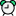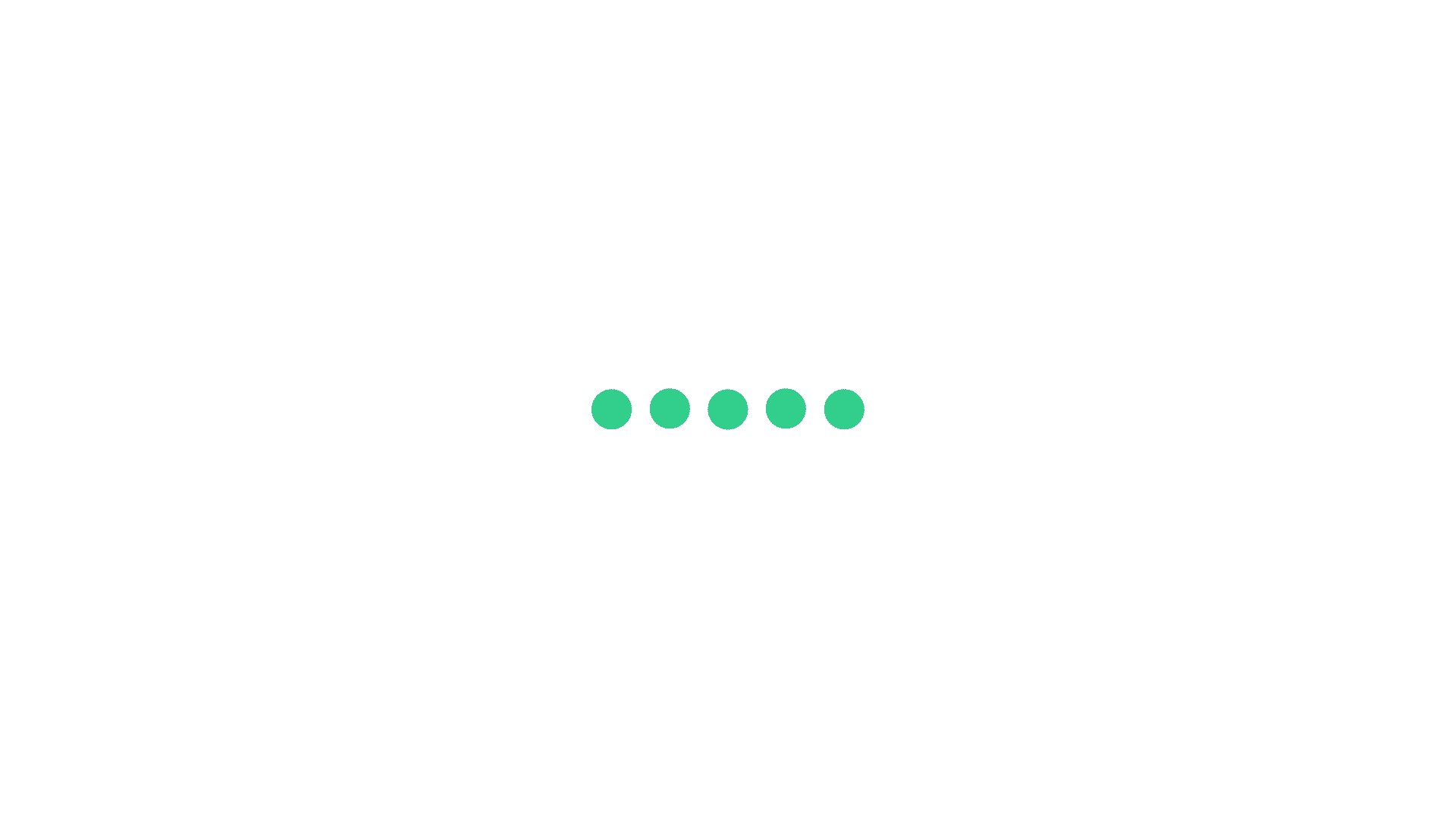# TCS Divisibility Question Answer 4

Question 1Time: 00:00:00
Number when successively divided by 5, 3, 2 gives remainder 0, 2, 1 respectively in that order. What will be the remainder when the same number is divided successively  by 2, 3, 5 in that order?4, 3, 2

4, 3, 2

1, 0,4

1, 0,4

2, 1, 3

2, 1, 3

4, 1, 2

4, 1, 2

Once you attempt the question then PrepInsta explanation will be displayed.Start

Question 2Time: 00:00:00
a,b,c,d,e are distinct numbers. if (75-a)(75-b)(75-c)(75-d) (75-e)=2299 then a+b+c+d= ?292

292

119

119

339

339

129

129

Once you attempt the question then PrepInsta explanation will be displayed.Start

Question 3Time: 00:00:00
265x is divisible by 5 then value of x will bezero or 5

zero or 5

5

5

zero

zero

7

7

Once you attempt the question then PrepInsta explanation will be displayed.Start

Question 4Time: 00:00:00
How many 9 digit numbers are possible by using the digits 1, 2, 3, 4, 5 which are divisible by 4 if the repetition is allowed?$5^{8}$

$5^{8}$

$4^{7}$

$4^{7}$

$6^{9}$

$6^{9}$

$7^{2}$

$7^{2}$

Once you attempt the question then PrepInsta explanation will be displayed.Start

Question 5Time: 00:00:00
What is the lowest possible integer that is divisible by each of the integers 1 through 7, inclusive?411

411

420

420

470

470

658

658

Once you attempt the question then PrepInsta explanation will be displayed.Start

Question 6Time: 00:00:00
If N=4P, where P is a prime number greater than 2, how many different positive even divisors does n have including n?4

4

6

6

8

8

5

5

Once you attempt the question then PrepInsta explanation will be displayed.Start

Question 7Time: 00:00:00
What is the remainder when 617+1176 is divided by 7?1

1

6

6

3

3

zero

zero

Once you attempt the question then PrepInsta explanation will be displayed.Start

Question 8Time: 00:00:00
The remainder when m + n is divided by 12 is 8, and the remainder when m - n is divided by 12 is 6.  If m > n, then what is the remainder when mn divided by 6?3

3

4

4

2

2

1

1

Once you attempt the question then PrepInsta explanation will be displayed.Start

Question 9Time: 00:00:00
X = 101102103104105106107......146147148149150

(From numbers 101-150).

Find out the remainder when this number is divided by 9.1

1

2

2

3

3

4

4

Once you attempt the question then PrepInsta explanation will be displayed.Start

Question 10Time: 00:00:00
Two positive number differ by 2001.When the larger number is divided by the smaller number, the quotient is 9 and the remainder is 41. The sum of the digits of the larger number is:11

11

14

14

13

13

15

15

Once you attempt the question then PrepInsta explanation will be displayed.